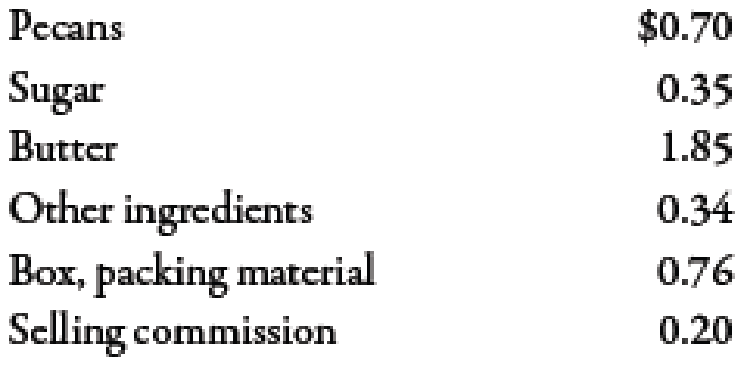Chapter 7, Problem 62P### Managerial Accounting: The Corners...

7th Edition
Maryanne M. Mowen + 2 others
ISBN: 9781337115773

#### Solutions

Chapter
Section### Managerial Accounting: The Corners...

7th Edition
Maryanne M. Mowen + 2 others
ISBN: 9781337115773
Textbook Problem
58 views

# Candyland Inc. produces a particularly rich praline fudge. Each 10-ounce box sells for $5.60. Variable unit costs are as follows:Fixed overhead cost is$32,300 per year. Fixed selling and administrative costs are $12,500 per year. Candyland sold 35,000 boxes last year.Required: 1. What is the contribution margin per unit for a box of praline fudge? What is the contribution margin ratio? 2. How many boxes must be sold to break even? What is the break-even sales revenue? 3. What was Candyland’s operating income last year? 4. What was the margin of safety in sales dollars? 5. CONCEPTUAL CONNECTION Suppose that Candyland Inc. raises the price to$6.20 per box but anticipates a sales drop to 31,500 boxes. What will be the new break-even point in units? Should Candyland raise the price? Explain.

1.

To determine

Calculate contribution margin for a box of praline fudge. Also calculate the contribution margin ratio.

Explanation

Contribution margin:

Contribution margin can be defined as the amount obtained after deducting the variable expense from sales revenue. It means the amount of sales left after covering the variable expenses.

Contribution Margin Ratio:

The sales percentage remaining after covering the amount of total variable cost is known as the contribution margin ratio. It is the available sales dollar percentage which will be used to cover the total fixed cost.

Use the following formula to calculate contribution margin:

Contributionmarginperunit=SellingpriceperunitVariablecostperunit

Substitute $5.60 for selling price per unit and$4.201 for variable cost per unit in the above formula.

Contributionmarginperunit=$5.60$4.20=$1.40 Therefore, the contribution margin per unit is$1.40.

Use the following formula to calculate contribution margin ratio:

Contribution margin ratio=Contribution marginperunitSellingpriceperunit

Substitute \$1

2.

To determine

Compute the break-even point in units and sales dollars.

3.

To determine

Calculate last year’s operating income of Company CI.

4.

To determine

Calculate margin of safety in sales dollars.

5.

To determine

Calculate the new break-even point in units after the change in price. Also recommend that the price should be increased or not.

### Still sussing out bartleby?

Check out a sample textbook solution.

See a sample solution

#### The Solution to Your Study Problems

Bartleby provides explanations to thousands of textbook problems written by our experts, many with advanced degrees!

Get Started

#### Find more solutions based on key concepts